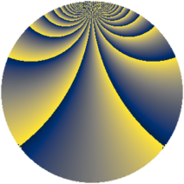# Properties

 Label 2736.2.yLevel $2736$ Weight $2$ Character orbit 2736.y Rep. character $\chi_{2736}(1331,\cdot)$ Character field $\Q(\zeta_{4})$ Dimension $288$ Sturm bound $960$

# Related objects

## Defining parameters

 Level: $$N$$ $$=$$ $$2736 = 2^{4} \cdot 3^{2} \cdot 19$$ Weight: $$k$$ $$=$$ $$2$$ Character orbit: $$[\chi]$$ $$=$$ 2736.y (of order $$4$$ and degree $$2$$) Character conductor: $$\operatorname{cond}(\chi)$$ $$=$$ $$48$$ Character field: $$\Q(i)$$ Sturm bound: $$960$$

## Dimensions

The following table gives the dimensions of various subspaces of $$M_{2}(2736, [\chi])$$.

Total New Old
Modular forms 976 288 688
Cusp forms 944 288 656
Eisenstein series 32 0 32

## Trace form

 $$288q + O(q^{10})$$ $$288q + 16q^{16} + 80q^{22} + 48q^{28} - 64q^{34} - 144q^{40} + 64q^{43} + 288q^{49} + 16q^{52} + 128q^{55} - 48q^{58} + 32q^{67} - 80q^{82} - 16q^{88} - 96q^{91} - 48q^{94} + O(q^{100})$$

## Decomposition of $$S_{2}^{\mathrm{new}}(2736, [\chi])$$ into newform subspaces

The newforms in this space have not yet been added to the LMFDB.

## Decomposition of $$S_{2}^{\mathrm{old}}(2736, [\chi])$$ into lower level spaces

$$S_{2}^{\mathrm{old}}(2736, [\chi]) \cong$$ $$S_{2}^{\mathrm{new}}(48, [\chi])$$$$^{\oplus 4}$$$$\oplus$$$$S_{2}^{\mathrm{new}}(144, [\chi])$$$$^{\oplus 2}$$$$\oplus$$$$S_{2}^{\mathrm{new}}(912, [\chi])$$$$^{\oplus 2}$$